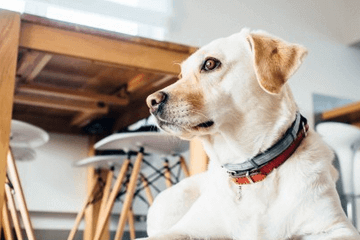洋气又招财的小狗名字Lucky
Lucky指幸运的意思，指狗狗与主人相遇非常幸运，狗狗有家，主人有陪伴得之所幸之意，且名字融入了西方文化也能彰显出主人的文化底蕴，而用lucky为狗狗取名也是非常的洋气好听，叫起来的顺口悦耳，使其在生活中幸运和财运的意思。1、选取与财字相关的字词取名

2、中西结合法

3、选取与财运的事物进行参照

4、使用叠词取名1、{多多}{温莉}{丢丢}{吉利}

2、{双双}{艾菲}{东东}{一筒}

3、{白白}{奥奇}{菲丽}{库仑}

4、{哈利}{小菲}{岁岁}{福生}

5、{宁宁}{将军}{麻团}{大帅}

6、{小黑}{米菲}{诺雅}{来宝}

7、{米莱}{菲菲}{拍拍}{进宝}

8、{亚梦}{奶昔}{毛毛}{满满}

9、{冰沙}{馒头}{小白}{公主}

10、{茉莉}{拉登}{小灰}{荣华}

11、{威廉}{王子}{麦琪}{辛巴}

12、{雅琦}{大款}{贝露}{洋洋}

13、{提拉}{坦克}{小福崽}{小Q}

14、{朵朵}{莫莫}{小榴莲}{阿贵}

15、{仔仔}{西蒙}{呆呆}{了了}

16、{丹尼}{水晶}{俊俊}{查理}

17、{BiBi}{卢布}{泪泪}{索菲亚}

18、{名牌}{元宝}{贝利}{贝克}

19、{美美}{多米}{樱木}{塞塞}

20、{哈里}{吉祥}{哈尼}{摩比}

21、{雪郎}{富贵}{罗特}{爱米}

22、{豆儿}{钱雨}{念念}{棉花}

23、{能能}{雷欧}{咪咪}{艾力克}

24、{乐乐}{轩轩}{星比}{doudou}

25、{小乖}{阿宝}{吉米}{豆沙包}

26、{果果}{道格}{豆丁}{伯爵}

27、{可乐}{花花}{佳鑫}{米粒QD}

28、{跳跳}{红双喜}{叮叮}{腻腻}

29、{叮当}{好运来}{牛牛}{香奈儿}

30、{哗啦}{胖胖}{衫衫}{黄金球}

31{天赐}{瑞瑞}{旺财}{吐吐}

32、{贝勒}{梦梦}{大赖}{怪怪}

33、{万福}{圈圈}{奔奔}{嘿嘿}

34、{如意}{贝塔}{贝贝}{皮卡}

35、{旺旺}{mary}{玛雅}{Luck}

36、{雅虎}{丽莎}{白板}{球球}

37、{麒麟}{百合}{幸运}{枫枫}

38、{港币}{托尼}{发菜}{大奔}

39、{啊萨}{皮皮}{法郎}{奇奇}

40、{笨笨}{卡卡}{米雅}{杰西卡}

41、{圆圆}{摩卡}{蛋蛋}{杰酷比}

42、{洛洛}{比力}{飞飞}{棉花糖}

43、{悠悠}{ 宝如}{豆豆}{索丽亚}

44、{小治}{米苏}{天狼}{冰欺凌}

45、{当当}{想想}{巴克}{莉莉斯}

46、{咖啡}{囔囔}{金金}{leobaby}

47、{肥婆}{鼎鼎}{兜兜}{巴洛克}

48、{宾宾}{肥肥}{福娃}{嘟嘟}

49、{小兰}{狗狗}{钻石}{奶黄包 }

50、{崽崽}{饭团}{貔貅}{BOSS}

51、{酷酷}{傻傻}{金条}{紫罗兰}

52、{璐璐}{妮妮}{鲁卡}{迪迪}

53、{格格}{哈哈}{瑶瑶}{苏苏}

54、{安迪}{帅哥}{哈瑞}{土豪金}

55、{点点}{富饶}{三宝}{汉堡包}

56、{路德}{小卒}{旺旺}{胜胜}

57、{房东}{西迷}{柯尔}{亮哥}

58、{瑞克}{摩卡}{地主}{泡泡}

59、{疯子}{五魁}{小宝}{西西}

60、{龙龙}{小鱼}{馒头}{痒痒}

61、{巴克}{帅虎}{小榆}{曦曦}

62、{哈瑞}{黑仔}{小派}{憨皮}

63、{元宝}{兜兜}{斯玛}{名名}

64、{木木}{哎呦}{毛毛}{双双}

65、{东东}{咕噜}{小武}{米酒}

66、{皇子}{尧尧}{寻乐}{ojbk}

67、{兰克}{老爷}{多多}{波波}

68、{跳跳}{点点}{学学}{小狼}

69、{大宝}{挠挠}{晨晨}{小凡}

70、{楠楠}{帅哥}{老大}{白虎}

71、{凯琳}{优卡}{阿旺}{噜噜}

72、{特工}{莫莫}{老板}{旺财}

73、{路飞}{西蒙}{奇奇}{大牛}

74、{刺猬}{阿萨}{棒棒}{睿哥}

75、{煤球}{来福}{拍拍}{蜘蛛}

76、{威风}{尼可}{小雪}{源源}

77、{阿虎}{小尘}{上上}{金秋}

78、{长毛}{小莫}{卡卡}{小黑}

79、{浣熊}{毛线}{绯绯}{香槟}

80、{胖墩}{胡圈}{二傻}{啤酒}

81、{堡扑}{能能}{航航}{风纪}

82、{肉包}{巴马}{嘻嘻}{迪迪}

83、{咖啡}{骆驼}{昊昊}{哈利}

84、{上帝}{大锤}{狗娃}{小治}

85、{凌云}{狂风}{大爷}{狮王}

86、{始源}{公子}{鹏鹏}{天王}

87、{图图}{布丁}{啊冰}{黑风}

88、{五爪}{墨墨}{黑客}{疾风}

89、{小智}{果汁}{小轩}{圣诞}

90、{熊喵}{璐璐}{小强}{旋风}

91、{蛋蛋}{小峻}{罗杰}{将军}

92、{闪电}{小源}{壮壮}{捕头}

93、{小木}{小誉}{小哈}{亮亮}

94、{小小}{洋洋}{黑虎}{肉团}